﻿ 几道水题的题解 – Hzao's Blog

# 几道水题的题解

### 逆序排列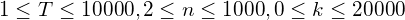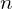的全排列有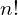个，每个测试点的数据组数很多。考虑动态规划。

1296 有限制的排列 一样，假如以“当前考虑到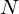的全排列的第位，且逆序对数为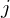的排列个数”来定义状态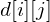，必然会困于如何去重。即使找到去除重复数字的方法，也会导致时间复杂度偏高。

1. 将原排列中，大于等于的数加上1；
2. 在最后添上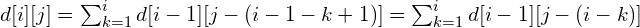#include<cstdio>#include<algorithm>#include<cstring>using std::sort;const long long maxn=1000,mod=1e9 + 7;long long n,k,done=1;long long ans[10*maxn+5];long long sum;struct qst{    long long n,k,id;}Q[maxn*10+5];inline void read(long long &x){    x=0;char c;long long f=1;    do{        c=getchar();if(c=='-')f=-1;    }    while(c>'9'||c<'0');    do{        x=x*10+c-'0';c=getchar();    }    while(c>='0'&&c<='9');    x*=f;}inline long long max(long long a,long long b){    return a>b?a:b;}long long d;void work(){    d=1;    sum=1;    for(register int i=1;i<=k;++i){        sum[i]=1;    }    for(register long long i=1;i<=n;++i){        for(register long long nk=0;nk<=k;++nk){            d[i&1][nk]=0;            d[i&1][nk]=(sum[(i-1)&1][nk]-(nk-i>=0?sum[(i-1)&1][nk-i]:0)+mod)%mod;            if(i==Q[done].n&&nk==Q[done].k){                ans[Q[done++].id]=d[i&1][nk];            }            sum[i&1][nk]=((nk?sum[i&1][nk-1]:0)+d[i&1][nk])%mod;        }    }}inline bool cmp(qst a,qst b){    if(a.n!=b.n)return a.n<b.n;    return a.k<b.k;}int main(){    long long T;    read(T);    for(register long long i=1;i<=T;++i){        read(Q[i].n);        read(Q[i].k);        n=max(n,Q[i].n);        k=max(k,Q[i].k);        Q[i].id=i;    }    sort(Q+1,Q+1+T,cmp);    work();    for(register long long i=1;i<=T;++i){        printf("%lld\n",ans[i]);    }    return 0;}

### 最长递增路径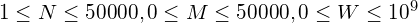1.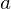的终点是的起点。
2.的长度必须严格小于的。

#include<cstdio>#include<algorithm>#include<cstring>using std::sort;const int maxn=5e4;struct line{    int u,v,w;}l[maxn*2+5];int ans;int d[maxn*2+5],maxb[maxn+5];inline void read(int &x){    x=0;char c;int f=1;    do{c=getchar();if(c=='-')f=-1;}while(c>'9'||c<'0');    do{x=x*10+c-'0';c=getchar();}while(c>='0'&&c<='9');x*=f;}inline bool cmp(line a,line b){    return a.w<b.w;}int n,m;int main(){    read(n);read(m);    for(register int i=1;i<=m;++i){        read(l[i].u);read(l[i].v);read(l[i].w);        l[i+m].w=l[i].w;        l[i+m].u=l[i].v;        l[i+m].v=l[i].u;    }    sort(l+1,l+1+2*m,cmp);    int lst=1;    for(register int i=1;i<=2*m;++i){        if(l[i].w!=l[lst].w){            for(register int j=lst;j<i;++j){                maxb[l[j].v]=std::max(maxb[l[j].v],d[j]);            }            lst=i;        }        d[i]=maxb[l[i].u]+1;        if(d[i]>ans)ans=d[i];    }    printf("%d\n",ans);    return 0;}

### 选数字

1. 读入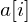时，假如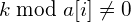，则直接忽略，读入下一个数字。
2.与之前已经生成的某个数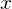构成新的数时，若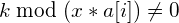则跳过。与已有的数来更新新的数时，应该将所有的修改都保存起来，等到更新完了之后再应用更新。什么意思呢？假如读入了一个数，之前已经有了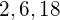这三个数，且构成这三个数的方案数分别为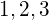。由我们的推算可知，加入后，可以由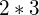来使构成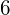的方案数加，由使构成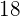的方案数加，由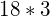使构成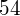的方案数加。加上本身，处理之后的已有数字和方案数分别为：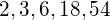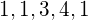。但是如果我们边处理边更新，会导致同一个被重复计算。给的方案数加1后，新增的这个已经用过的方案，又与一起更新了的答案。所以应该把每次要修改的操作保存起来，把所有的待更新数更新完毕后，再应用修改到方案数组中。

#include<cstdio>#include<map>using std::map;const long long maxn=1e3;const long long mod=1000000007;long long n,k;long long a[maxn+5];map<long long,long long>d;map<long long,bool> ment;long long mentd[maxn+5];struct addo{    long long to,val;    addo(long long to=0,long long val=0):to(to),val(val){}}add[maxn+5];inline void read(long long &x){    x=0;char c;long long f=1;    do{c=getchar();if(c=='-')f=-1;}while(c>'9'||c<'0');    do{x=x*10+c-'0';c=getchar();}while(c>='0'&&c<='9');x*=f;}void work(){    for(register long long i=1;i<=n;++i){        read(a[i]);        if(k%a[i]!=0)continue;        long long lmentd=mentd,tadd=0;        for(register long long j=1,y;j<=lmentd;++j){            if(k%(y=a[i]*mentd[j])!=0)continue;            add[++tadd]=addo(y,d[mentd[j]]);            if(!ment[y]){                mentd[++mentd]=y;                ment[y]=true;            }        }        for(register long long j=1;j<=tadd;++j){            d[add[j].to]+=add[j].val;            d[add[j].to]%=mod;        }        d[a[i]]++;        d[a[i]]%=mod;        if(!ment[a[i]]){            mentd[++mentd]=a[i];            ment[a[i]]=true;        }    }    printf("%lld\n",d[k]);}int main(){    long long T;    read(T);    while(T--){        read(n);read(k);        work();        if(T){           ment.clear();           mentd=0;        }    }    return 0;}

### 整数划分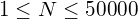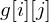表示用个数构成i的方案数，由于每个数各不相同，最多的数的个数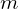满足。计算得知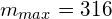个数字来组成，假如不选择数字，那么方案数为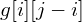，也就是把构成个数每个都加上；假如要选择数字，为了保证前面没有数字（题目要求所有数不相同），我们需要把前面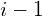个数加上1，再给最后一位放一个。方案数就是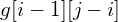（前位构成）。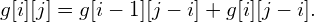#include<cstdio>
#include<cmath>
const long long mod=1e9+7;
const long long maxn=5e4;
long long g[maxn+5];
long long ans;
long long n,m;
int main(){
scanf("%lld",&n);
g=1;
for(register int i=1;i<=319;++i){
for(register int j=i;j<=n;++j){
g[i][j]=(g[i][j-i]+g[i-1][j-i])%mod;
}
}
for(register int i=1;i<=319;++i){
ans+=g[i][n];
ans%=mod;
}
printf("%lld\n",ans);
return 0;
}